# GO simulation in NAMD

## Hybrid MD-Go model

NAMD incorporates a hybrid MD-Go model (hereby referred to as Go) to study the conformation changes in biomolecular systems. The method replaces the physical-based nonbonded interactions with a smoother knowledge-based potential energy surface. Bonded interactions are taken from the classical force fields. By removing energetic traps along a MD trajectory, the system will be able to sample states not normally accessible to classical MD simulations.

## Hybrid MD-Go considerations

Typically, Go simulations are conducted in the absence of solvent and with electrostatic and van der Waals forces in the system turned off to improve conformational space exploration. Due to the current implementation of Go, the partial charges and van der Waals radii need to be set to zero in the psf and parameter file to remove the physical nonbonded interactions. Additionally, NAMD uses a reference PDB structure to construct the Go pairwise potential between atoms.

Finally, the Go model in NAMD introduces the idea of chain types. Consider modeling a protein-nucleic acid complex. Using classical all-atom MD, a single force field describes all possible nonbonded interactions. With Go, however, one can create separate nonbonded force fields to describe the protein and nucleic acid interactions. In order to create separate force fields, atoms are grouped together using chain types where the chain types are taken from the occupancy field of the reference PDB file. For argument sake, assume that the protein atoms have an occupancy value of 1.0 and that the nucleic acid atoms have an occupancy value of 2.0. One now must define three separate Go potentials for intra-protein, intra-nucleic acid, and inter-protein-nucleic acid interactions. In terms of chain types, this corresponds to (1) between atom pairs fully in chain 1, (2) between atom pairs fully in chain 2, (3) between atom pairs where one atom is in chain 1 and the other atom is in chain 2 respectively. To run Go, a minimum of one chain type must be defined.

## Configuration file modifications

The following configuration parameters are used to setup and run a Go simulation:

• GoForcesOnAre Go forces turned on?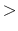Acceptable Values: on or off
Default Value: off
Description: Specifies whether or not Go forces should be calculated. If turned off', Go forces will not be calculated. If turned on', Go forces will be calculated. By default, the Go forces will be calculated in addition to the electrostatic and van der Waals forces. To simulate a system using only Go forces, the partial charges and Lennard-Jones parameters can be set to zero in the force field files.
• GoParametersParameter file defining Go potentialAcceptable Values: file
Description: File contains parameters to define the Go pairwise forces between different chain types. All possible chain type pairing combinations must be enumerated. Chain types are defined in the GoCoordinates file. The format for the GoParameters file is described in the next section.
• GoCoordinatesReference structure for Go simulationAcceptable Values: PDB file
Description: PDB file contains the reference structure used to define the Go potential. The file need not be the same file used to initialize the coordinates of the MD simulation; however, it must contain the same number of atoms in the same order as given in the structure (.psf) and coordinates (.coor) file. Additionally, the occupancy fields of the PDB file will be read to determine which chain type an individual atom belongs to, and, thus, which pairwise Go potential to use to calculate forces. By default, the occupancy value of 0.0 turns off the Go potential for that particular atom.
• GoMethodcontrols method for storing Go contact informationAcceptable Values: lowmem or matrix
Description: Specifies whether the Go contacts should be calculated on the fly or stored in a matrix respectively. In most cases, lowmem'will be sufficient. However, for smaller systems, the matrix' does offer a slight performance speedup in terms of wall time. Variable is only used if GoForcesOn is on'

The following sections describe the format of the GoParameter file.

## GoParameter format

When running a Go simulation, the atoms are partitioned into chains according to the occupancy value given in the GoCoordinates file. For every possible pairwise combination between chains, a Go potential is defined by the following equations:

Letbe the pairwise distance between atoms i and j in the reference structure. Ifis less than the Go cutoff distance, the pairwise potential between atoms i and j is given by:whereis given as. Ifis greater than the Go cutoff distance, the pairwise potential between atoms i and j is given by: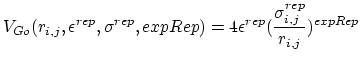For each pairwise chain combination, the following parameters are needed to define the Go potential:

• chaintypes (2 floats): (first_chain second_chain) Defines the pairwise chain interaction
• epsilon (1 float): () Determines theconstant of the Go potential in units of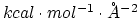• exp_a (1 integer): (a) Determines the a' constant for the Go potential
• exp_b (1 integer): (b) Determines the b' constant for the Go potential
• expRep (1 integer): (expRep) Determines the expRep' constant for the Go potential
• sigmaRep (1 float): () Determines theconstant for the Go potential in units of• epsilonRep (1 float): (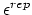) Determines theconstant for the Go potential in units of• cutoff (1 float): (cutoff) Defines the Go cutoff distance for this particular pairwise chain in units of• [Optional] restriction (1 integer): Determines if interactions between the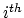andadjacent residue should be excluded. Multiple restriction between adjacent residues can be defined within a chaintype. Each additional new restriction is given on its own line.

Each pairwise chaintype should be written in its own block of text with each entry given its own line. It is recommended that individual pairwise potential be separated by a blank line.